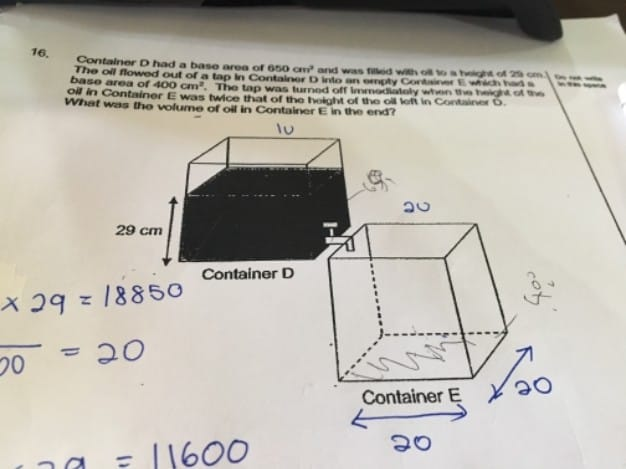# QuestionSource: 2017 sa2

Volume of oil in Container D = 29 × 650 = 18850 cm³

After the transfer of oil, let the height of the oil in Container D be 1 unit.

Then the height of the oil in Container E is 2 units.

650 × 1 unit + 400 × 2 units = 18850

1450 units = 18850

1 unit = 13

Height of oil in Container E = 2 units = 13 × 2 = 26 cm

Volume of oil in Container E = 400 × 26 = 10 400 cm³

0 Replies 0 Likes